# 3 Peristaltic pump flow calibration

3.1 Volumetric Calibration of the peristaltic pump flow
3.2 Calibration of pump flow rate by weight

A short video of peristaltic pump flow calibration can be found at www.lambda-instruments.com/peristaltic-pumps/#video.

The pump speed is proportional to the flow. By determining the total amount of liquid delivered at a given pumping speed over a measured time interval (duration), you can calibrate the flow rate.

Before calibration the pump flow rate, make sure that the liquid completely fills the pump tubing and reaches its free end. Only then initiate the measuring process, for precise calibration.

The calibration of the pump flow rate with speed can be done to know the amount of the liquid pumped.

#### Two methods to calibrate the flow rate of peristaltic pumps could be found below:

1. Volumetric calibration of pump flow rate
2. Calibration of pump flow rate by weight

## 3.1 Volumetric calibration of the peristaltic pump flow

In the volumetric calibration, the amount of liquid pumped at a particular speed for a minute is calculated. Program the pump with flow rate say 600 and time of about 1 minute.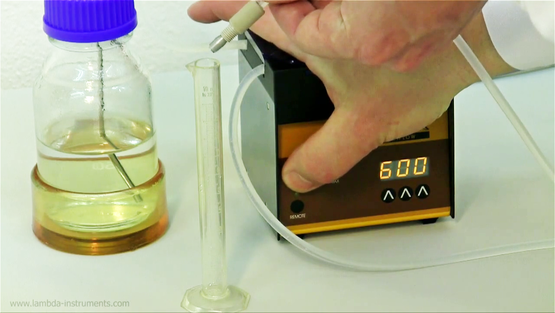Figure 3.1-1 Turn on the pump and have the other end of the tubing ready near the measuring cylinder. Carefully collect the liquid being pumped in the measuring cylinder for about 60 seconds.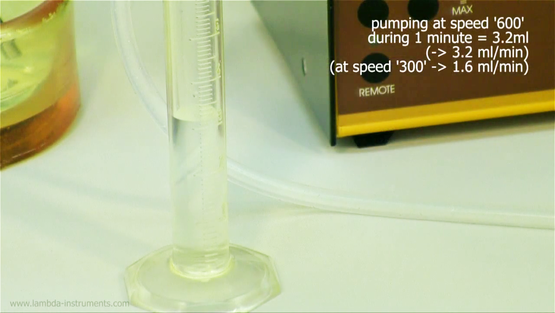Figure 3.1-2 Measure the volume of liquid collected in a minute. At the speed of 600, 3.2 ml/minute has been collected. The flow rate for other speed range can be calculated using this value.

## 3.2 Calibration of pump flow rate by weight

In the calibration by weight, the weight of the liquid pump in a minute was calculated. Program the MULTIFLOW, HIFLOW, MAXIFLOW and MEGAFLOW peristaltic pumps for the calibration by weight.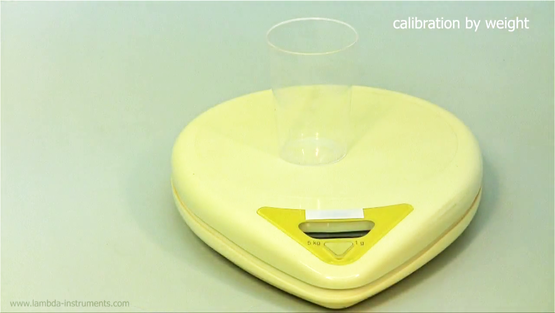Figure 3.2-1 Measure the weight of the empty beaker using a sensitive weighing scale. For the exact measurement of the liquid collected, tare the weighing scale with the measuring beaker (Example: 0.000 g).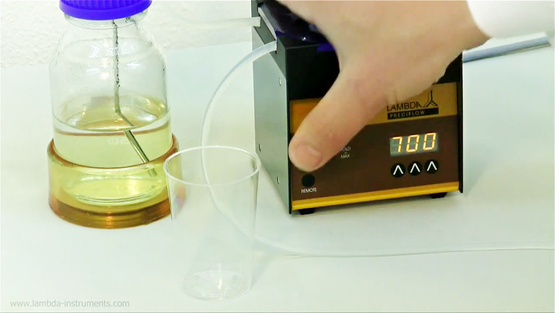Figure 3.2-2 Connect the one end of the tubing to the liquid source and press ON/OFF (PRECIFLOW pump) or RUN button of the peristaltic pump to start the program (MULTIFLOW, HIFLOW, MAXIFLOW and MEGAFLOW pumps).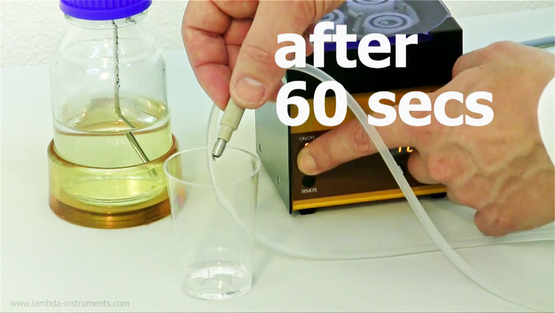Figure 3.2-3 Carefully collect the liquid being pumped into the measuring beaker for about 60 seconds. Pump gets switched off exactly after 60 seconds as programmed.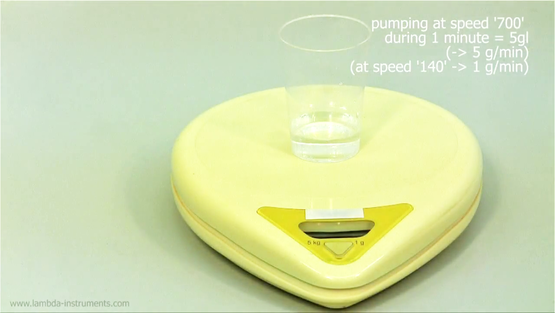Figure 3.2-4 Weigh the measuring beaker with the liquid been collected in the past 60 seconds. For example: At the pumping speed of 700, the weight of the liquid collected is 5 g/min. With this calculation, the weight of liquid collected at other pump speed can be found.